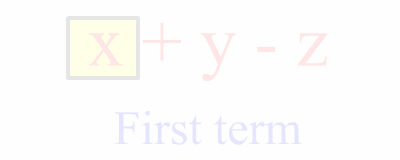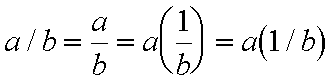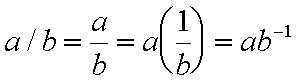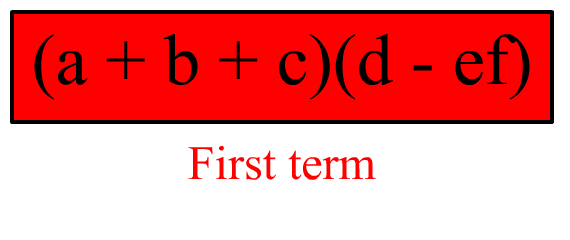# Expression Evaluation, Terms and Factors

When discussion expression evaluation it is handy to understand what is meant in mathematics by the words 'term' and 'factor'.

We have looked at expressions and discussed them from the viewpoint of operators and operands. Now, we will look at an expression from the viewpoint of terms and factors. From this viewpoint we are less concerned with the interactions among the parts of the expression, but more concerned with how to generally name the parts of an expression.

Terms are those parts of the expression between addition signs and subtraction signs. Consider this expression:

x + y - z

This expression has three terms: the variables x, y, and z. We say that the first term is x, that the second term is y, and so on.Here is another expression:

7 - f(x) + a

This expression also has three terms: the number 7, the function f(x), and the variable a.

The first term is the number 7, the second term is the function f(x), and the third term is the variable a.

Now, consider this expression:

xy + 2

Here, the first term is this:

xy

Notice that this term is made up of two parts, the variable x and the variable y.

Factors are the separate parts of a multiplication. Consider this expression:

xy

The above, of course, is a multiplication of x times y. The factors in this multiplication are x and y.

In the following expression the factors are 8, p, and q:

8pqNow, back to this expression:

xy + 2

Here the first term is xy, and that first term is made up of two factors, x and y.

So, terms are groups of factors.

The second term in this expression is the number 2. This term is made up of one factor, 2.

Consider this expression:

cd2

This expression has one term which is made up of two factors. The first factor is c. The second factor is d2.

In other words, powers are considered single factors. The factor d2 is not considered two factors even though it is equal to d times d.

What about division? Well, it can defined in terms of multiplication, as in:So, one could say that the factors of a/b are a and 1/b.

Also, we could work things this way:And clearly see that the factors of a/b are a and b-1.

So, terms are inside of factors, but the factors themselves can be made up of terms which can have factors inside of them, and so it. It can get quite complicated! Consider this:

(a + b + c)(d - ef)

From the outer most viewpoint, this is an expression with one term, the entire expression.

From this outer most viewpoint the first and only term in this expression is made up of two factors. The first factor is:

(a + b + c)

The second factor of this first and only term is:

(d - ef)

Now, let's look at that first factor of the first term. Again it is:

(a + b + c)

Or simply:

a + b + c

This factor is itself made up of three terms: a, b, and c. Each of these terms has but one factor since each is but one variable.

Let's also look at the second factor of the first term. Again it is:

(d - ef)

Or simply:

d - ef

This factor is made up of two terms, d and ef.

The first term here, d, is made up of one factor, the single variable d.

The second term here is made up of two factors, e and f.

So, considering the original expression:

(a + b + c)(d - ef)

One could correctly say that the second term of the second factor is ef.

Taking it just a bit further, the second factor of the second term of the second factor of the original expression is f.

And so on...Where are you?

Here: Terms and Factors

Custom Search